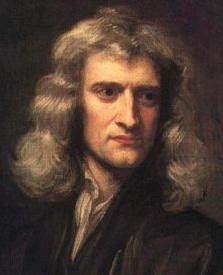Isaac Newton
(1643 - 1727)
 The founding fatherofMathematical Physics
The mathematical establishment holds Newton up as a god for allegedly having discovered calculus, the Three Laws of
Motion, and the Universal Law of Gravitation. Actually, Newton fails the three distinctions.

1. Calculus

The mainstream gives the wrong impression that we were living in the dark ages and that suddenly a bright fellow named
Newton came along and out of nowhere discovered or invented the mathematical technique called calculus. This is
certainly false. The objective facts are that Newton published his version of calculus in 1687, more than 10 years after
Leibniz. Nevertheless, the calculus was developed gradually by a body of mathematicians that exchanged ideas no
differently, I may add, than the team that developed the transistor. A few of the mathematicians that participated in this
process were Gauss, Descartes, and Huygens. Most of these mathematically-inclined individuals traveled and talked to
each other and read each others' manuscripts. Despite the difficulty of traveling and low level of communication (compared
to today's Internet), there was nonetheless feedback in those days. It was in this environment that the idea of calculus
flourished. The development of calculus was a concerted effort, similar to the development of the atom bomb or the TV or
the chip. As you would expect, more sophisticated versions of calculus surfaced with the passage of time. We can perhaps
credit Newton with making a strategic breakthrough like Shockley made with the transistor, but this doesn't invalidate other
contributions to this intergenerational effort.

2. The three 'laws' of motion

Newton is also credited with discovering the three laws of motion. The first one says that you will travel in a straight line
unless someone bumps you. The third one say that an action generates a reaction. You push the bully and instantly feel
some kind of pain. But it is the second law which really made him famous. This law, allegedly discovered by Huygens and
reformulated by Newton, says that force is equal to mass times acceleration (F = ma).

Unfortunately, Newton failed to follow the scientific method and his three alleged 'laws' end up in the trash can. Specifically,
Newton failed to define the word motion. In fact, he took for granted that you already knew what this strategic word meant:

“ I do not define time, space, place and motion, as being well known to all.” (p. 81) 

[No kidding?]

Then, he nevertheless gave it his best shot:

“ Absolute motion is the translation of a body from one absolute place to another;
and relative motion, the translation from one relative place into another.” 

[Great Newt! Motion is the translation and the translation is motion. Is this what the mathematicians are referring to
when they say that Mathematical Physics is founded upon 'rigorous definitions'?]

Newton's first 'law' fails because no object in the Universe may travel rectilinearly, let alone straight, for I ask, with respect
to what? Can anything travel rectilinearly with respect to Jupiter or the Moon? The 2nd 'law' is of concern only to
Mathematics. It is a technique to calculate an amount and, thus, has nothing to do with Physics. Like all other formulas
and equations of Mathematics, the expression (F=ma) is just a description that relies on still undefined terms such as
mass and acceleration. A description without more is not Science. Until Newton gives a physical interpretation of these
terms (i.e., expresses them in terms of physical objects) his formula is worthless. As of today, the idiots of Mathematics
have no idea what the words force, mass, and acceleration mean in terms of physical objects. The third 'law' -- I push
you and you push back -- is not a law, but a trivial fact. Newton has yet to explain the reason for inertia. What causes
gravity, Newt? What is mass?

3.   The Universal Law of Gravitation

If relativists have their way, Newton's contributions to our current knowledge of gravity and gravitation are immaterial.
According to relativity, Newton's explanation for gravity is ludicrous because it relies on forces and particles. The
establishment has now allegedly confirmed through experiments such as Gravity Probe B that space is warped. There is
nothing more to argue. Likewise, the mainstream doctrine is that Einstein's equation, which has no resemblance to
Newton's other than in the petty low velocity scenario, is correct, and Newton's formula should be discarded. Newton's
entire theory has been upstaged by Einstein's General Relativity.

To make matters worse, Newton had no explanation for why his gravitation equation works. "Hypothesis non fingo" he

" That one body may act upon another at a distance through a vacuum, without the
mediation of any thing else, by and through which their action and force may be
conveyed from one to the other, is to me so great an absurdity, that I believe no
man who has in philosophical matters a competent faculty of thinking, can ever
fall into it." 

Therefore, if relativists are correct, Newton shouldn't get any credit for his efforts. His work was in vain.

And then again you wonder why the people at NASA use Newton's force model and gravitational equation and not
Einstein's when they know that Newton's physical interpretation is incorrect. Why does Newton's gravitational equation
work if it invokes a totally different physical mechanism? This is what the idiots of Mathematical Physics have to answer
instead of spending time and journal space on nonsense such as time travel and annihilation and Gravity Probe B.

________________________________________________________________________________________